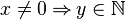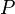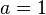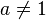# Proof Hints

## Introduction

This document does NOT provide a way to avoid doing interactive proofs or pointing out the weakness of existing provers. Rather this document shows how to use information from doing interactive proofs to improve the clarity of models. As a consequence of these improvement, more obligations can be discharged automatically. Below are our proposal for two kinds of improvements: hypothesis selection and case distinction.

## Improvements

The presentation for each kind of improvement is as follows:

1. Description: A brief description of the proof hints with the situation where this can be helpful.

2. Example: an example in Event-B is presented.

3. Work around: we show a solution (rather a work-around) for the current RODIN Platform.

### Hypothesis Selection

Description In order to discharge the proof obligation, the developer just selects some hypotheses then invoke either AtelierB P0 or newPP restricted to finish these proofs. The solution is to (somehow) give "hints" to the Proof Obligation Generator (POG) to select these hypotheses automatically when generating the corresponding proof obligation.

Example Consider the following Event-B model

Consider the proof obligation set/hypSel0_1/INV which states that the event set maintains invariant hypSel0_1 (Here$\ldots$ denotes the unselected hypotheses).Any provers works with only the selected hypotheses cannot discharge the above proof olbligation since the hypothesis$x \neq 0 \limp y \in \nat$ is missing. The obligation with the appropriate hypothesis selection is as followsWorkaround Currently, given and event evt and invariant inv, the invariant preservation proof obligation evt/inv/INV generated with the following hypotheses automatically selected;

• The invariant inv
• The guard of the event evt

In order to have the needed hypothesis selected automatically, we will add this hypothesis into the guard. Moreover, in order to avoid changing event's behaviour, we will mark this extra guard as a theorem. Apply this technique, the new event set is as followsNote that the extra (theorem) guard can be removed in the further refinement without generating any new proof obligation.

### Case distinction

Description In order to discharge a proof obligation, the developer makes a manual "proof by cases" based on some condition$P$. Afterwards, all branches of the proofs are discharged either by ML or AtelierB P0. The solution is to (somehow) give "hints" to the POG to generate different proof obligations for each case separately. Proof by cases is usually not applied automatically since this splits the proof tree which could lead to blows up in terms of the number of cases.

Example Consider the following Event-B model

Consider the proof obligation set/case0_1/INV (Here all hypotheses, including those hidden are shown)

In order to finish the proof, a correct "proof by cases" is done to consider$a = 1$ or$a \neq 1$.

Workaround To capture the effect of doing proof by cases, we split the original event set into two different events as follows

In the next refinement step, we can merge the above two new event to the original event as follows.